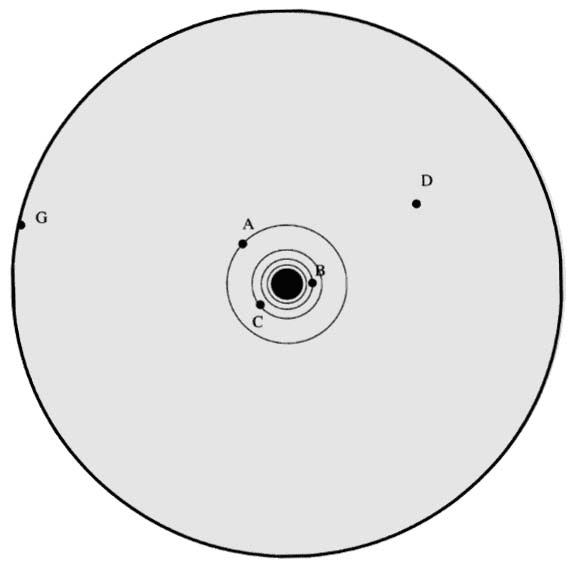# Electric Field and Force

dimpledur

## Homework StatementA 12 V battery gets connected between the center conductor (black dot) and the sheath of the coaxial cable so that the voltage of the center conductor with respect to the sheath (outer contour) is +12 V. What is the approximate force on a +25C particle located at D.

## Homework Equations

$$F=(Vq)/x$$

## The Attempt at a Solution

Electric field lines extend from the center conductor to the sheath, with field vectors pointing from the center to the outer contour.

Would the average force then just be $$F=(+12V*+12C)/x$$

and x would merely be measured from the center conductor to the position D?

We're told the radius of the circle is 1cm.

## Answers and Replies

Mentor
Note that, if you imagine the electric field lines between the inner and outer conductor, that they diverge as they get further from the center. That means that the field won't be a uniform magnitude over all distances as it is with parallel plates.

Have you used Gauss' law before? You should be able to discover the mathematical form of the electric field between the conductors using Gauss' law.